Electronic Circuit Simulation - Static Analysis Implementation (part 2) [Python]
[Custom Thumbnail]

All the Code of the series can be found at the Github repository:
https://github.com/drifter1/circuitsim

Introduction

Hello it's a me again @drifter1! Today we continue with the Electronic Circuit Simulation series, a tutorial series where we will be implementing a full-on electronic circuit simulator (like SPICE) studying the whole concept and mainly physics behind it! In this article we continue with the Implementation of a complete simulator for Static Analysis. The second step has to do with the mapping of the various "string" nodes into indexes of the MNA Matrices, which is something that can be done using a Hash table...

Requirements:

• Physics and more specifically Electromagnetism Knowledge
• Knowing how to solve Linear Systems using Linear Algebra
• Some understanding of the Programming Language Python

Difficulty:

Talking about the series in general this series can be rated:
Today's topic(s) can be rated:
• Intermediate

Actual Tutorial Content

In the MNA System's Matrices each row and column corresponds to a specific node of the Electronic Circuit. But, because the high and low terminals/nodes in the Netlist are strings and so not always directly numbers (or even when they are numbers, they might not be in a closed range starting from index 0), we will have to map those strings into specific integers in the range [0, nodeCount - 1]. We don't know where exactly each node occurs in the Netlist beforehand, and so we will have to store the string-integer relation in some kind of structure.

Which structure does that remind you of? Well, that's quite simple I think! We need a Dictionary, which is basically a Hashtable.

Node Hashtable

For our node mapping problem we have:
• The index or key of each item in the Dictionary is basically the "string" node that we want to map into an integer
• The value of each item gives us the integer mapping that corresponds to each node

To be able to set the correct node-value we have to define a counter variable that increments whenever a new node is added to the Hashtable. That way the mapped value of the node-string will be either equal to:
• the value that already exists in the Hashtable
• the nodeCount (as we will increase it after adding)

After adding the node to the Hashtable and so after creating the new Item, we will return the value that is stored in the Hashtable, so that this value can be stored in the Component structure that we created last time. That way, we can easily use the high and low integer mappings of the high_str and low_str strings (tweaked the name from last time) to fill the MNA System!

The class of this Node Hashtable can be defined as:
class NodeHashtable:  # nodes hash table
def __init__(self):
self.nodes = {}
self.nodeCount = 0
def addToNodes(self, node_str): # check if node not already added if node_str not in self.nodes: self.nodes[node_str] = self.nodeCount self.nodeCount = self.nodeCount + 1 return self.nodes[node_str]
def __del__(self): del self.nodes

Let's explain each part of this class:
• In the initialization function "__init__()" we basically only define the dictionary/hashtable of nodes using "{}" and the counter variable nodeCount, that also gets initialized to 0.
• To add nodes to the Hashtable we define a new function "addToNodes()" that takes in a string-node and maps it into an integer, using the exact logic that we explained previously. In the end, this function also returns the mapped integer value. Also, let's not forget that we check if "node_str" was already mapped by checking if "node_str" is already a key of the nodes Dictionary.
• To delete the instance of such a class we also redefine the "__del__()" function, by simply calling the delete function for the nodes Dictionary.

Component Structure Modifications

Each component now has two more entries, which are the mapped integer values of the high and low string-nodes correspondingly. We will also change the name of the high and low strings from high_node and low_node to high_str and low_str, to not get confused! Doing that we of course have to change the occurrences of these variables in the representation function "__repr__()" too, to make this function print out the integer mappings of the nodes. So, in the end the new modified Component class is:
class Component:  # circuit component structure
def __init__(self, comp_type, high_str, low_str, value):
# component type
self.comp_type = comp_type
# nodes as strings
self.high_str = high_str
self.low_str = low_str
# mapped nodes
self.high = -1
self.low = -1
# component value
self.value = value
def __repr__(self): return str(self.comp_type) + " " + str(self.high) + " " + str(self.low) + " " + str(self.value)

And these are actually all the changes...

Node Mapping

Now that all that is out of the way, we can get into the main Node mapping part of this Tutorial! Node mapping is basically done using a simple function that loops through all the components of the components list that we created last time and sets the values of the high and low entries calling the "addToNodes" function with the high_str and low_str strings correspondingly. So, we only have to follow the steps:
1. create the Hashtable using the "NodeHashTable()" constructor
2. add the '0' node to make sure that it gets mapped to 0
3. loop through all the components and map their string-nodes into integers
4. return the components and hashtable

In Python code this looks like this:
def mapNodes(components):
# create hashtable
hashtable = NodeHashtable()
# for all components for component in components: component.high = hashtable.addToNodes(component.high_str) component.low = hashtable.addToNodes(component.low_str)
return components, hashtable

Let's now also tweak the main function to map the nodes and print out the node mappings. This looks like this in Code:
# Initialize component counters
voltageCount = 0
currentCount = 0
resistorCount = 0
capacitorCount = 0
inductorCount = 0
# Parse File fileName = "example.spice" print("Parsing file...\n") components = parseFile(fileName)
# Map nodes print("Mapping nodes...\n") components, hashtable = mapNodes(components)
# Print Information print("Circuit Info") print("Component count: ", len(components)) print("Voltage count: ", voltageCount) print("Current count: ", currentCount) print("Resistance count: ", resistorCount) print("Capacitance count: ", capacitorCount) print("Inductance count: ", inductorCount) print("Node count: ", hashtable.nodeCount)
print("\nNodes are mapped as following:") for key, val in hashtable.nodes.items(): print("\"" + key + "\" :", val)
print("\nCircuit Components:") for i in range(0, len(components)): print(components[i])

Running the new Code for the Example of last time we basically get the same strings as integers! The Console gives us: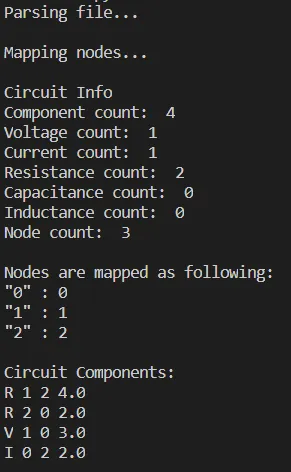But, this doesn't mean that something like that happens for every Netlist!

Circuit 1 - all nodes are numbers but get mapped differently

Consider the following Netlist:
V1 5 0 2
V2 3 2 0.2
V3 7 6 2
R1 1 5 1.5
R2 1 12 1
R3 5 2 50
R4 5 6 0.1
R5 2 6 1.5
R6 3 4 0.2
R7 7 0 1e3
R8 4 0 10
I1 4 7 1e-3
I2 0 6 1e-3
C1 7 0 0.1
C2 2 0 0.2
L1 12 2 0.1

Running our code for this Netlist we get: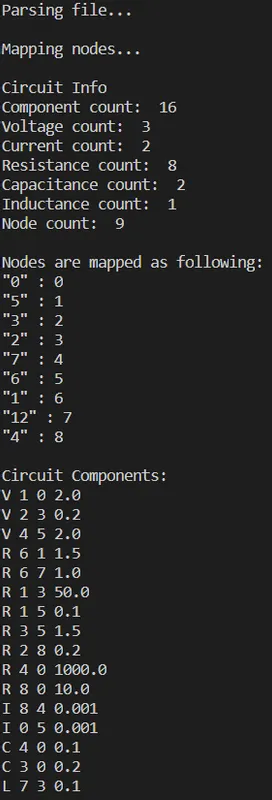I guess it's easy to see that the nodes were mapped quite "randomly". But it's not that random as it seems to be. The nodes just get the first value that is available when being mapped. Also, notice how there is a "12" node even though the nodes are actually only 9, or 8 if we forget about node '0'...

Circuit 2 - all nodes are strings

Consider the following Netlist:
R00 _n_00_00_ _n_25_00_ 4
R01 _n_25_00_ _n_50_00_ 32
R02 _n_00_00_ _n_00_25_ 18
R03 _n_25_00_ _n_25_25_ 15
R04 _n_50_00_ _n_50_25_ 92
R05 _n_00_25_ _n_25_25_ 15
R06 _n_25_25_ _n_50_25_ 28
R07 _n_00_25_ _n_00_50_ 33
R08 _n_25_25_ _n_25_50_ 29
R09 _n_50_25_ _n_50_50_ 12
R10 _n_00_50_ _n_25_50_ 48
R11 _n_25_50_ _n_50_50_ 90
Rg1 _n_25_00_ 0 100
Rg2 _n_50_00_ 0 100
Isrc _n_00_00_ 0 10

Running our code for this Netlist we get: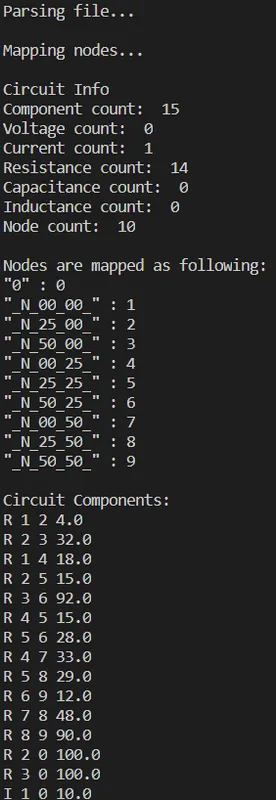Here you can more easily see how the node strings are turned into integers!

After that we only have to fill the MNA Matrices and solve the system...which is what we will do next time! Until now we basically did the following very important things with circuit components: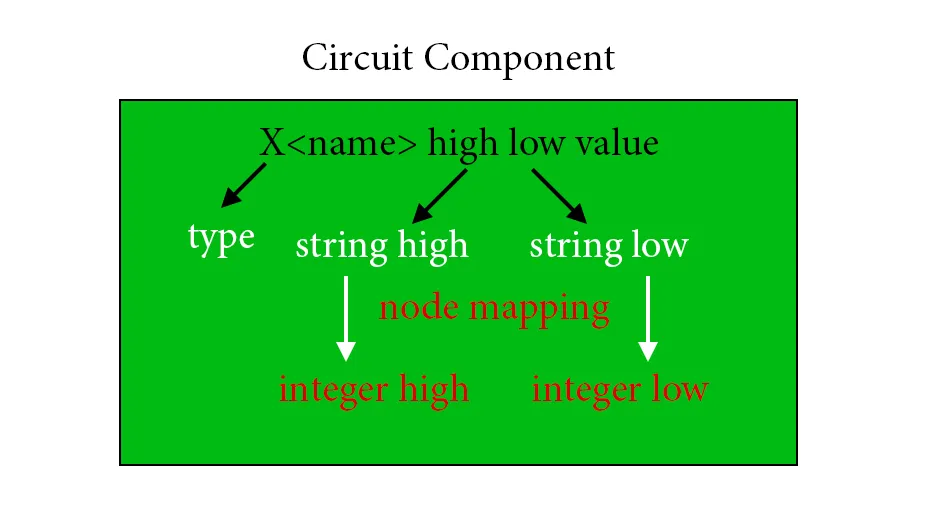RESOURCES

References:

1. https://www.w3schools.com/python/python_dictionaries.asp

Mathematical Equations were made using quicklatex

Final words | Next up on the project

And this is actually it for today's post and I hope that you enjoyed it!

Next time we will finish off the Implementation of the Electronic Circuit Simulator for Static Analysis, by filling and solving the MNA System...

So, see ya next time!

GitHub Account:

https://github.com/drifter1Keep on drifting! ;)

H2
H3
H4
3 columns
2 columns
1 column